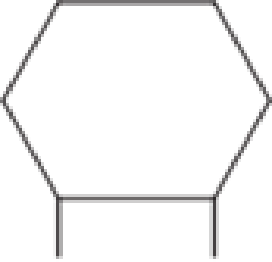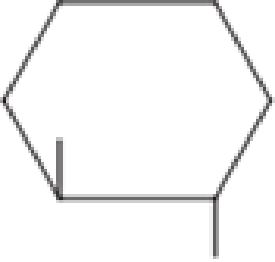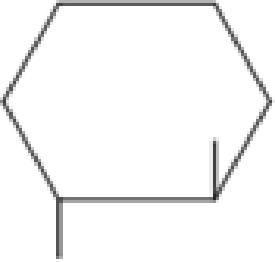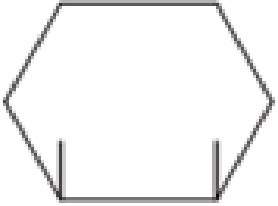Chapter 1, Problem 1.115EP### Organic And Biological Chemistry

7th Edition
STOKER + 1 other
ISBN: 9781305081079

#### Solutions

Chapter
Section### Organic And Biological Chemistry

7th Edition
STOKER + 1 other
ISBN: 9781305081079
Textbook Problem

# Classify as cis- or trans- the positioning of the alkyl groups in each of the following cycloalkanes.a.b.c.d.(a)

Interpretation Introduction

Interpretation:

Cis- or trans- positioning of the alkyl groups in the given cycloalkane has to be classified.

Concept Introduction:

Organic compounds are represented shortly by the molecular formula and structural formula.  Each and every compound has its own molecular formula.  Compounds can have same molecular formula but not same structural formula.

Isomers are the compounds that have same molecular formula but different structural formula.  The main difference lies in the way the atoms are arranged in the structure.  Isomers have different chemical and physical properties even when they have same molecular formula.  This is known as Isomerism.

If there is difference only in the connectivity of the atoms in the molecule, then it is known as constitutional isomerism.  The isomers are known as constitutional isomers.  They will have same molecular formula and same functional group, but they differ in the connectivity between the atoms in the molecule.

For constitutional isomers to be present in cycloalkane, a minimum of four carbon atoms has to be present.

Cycloalkanes can also exhibit stereoisomerism.  The difference between constitutional isomerism and stereoisomerism is that, the result of difference in connectivity of carbon atoms is known as constitutional isomerism and the result of differences in configuration is known as stereoisomerism.  Stereoisomers are compounds that possess same molecular formula and connectivity of atoms but different orientations of atoms in space.  Cis isomers are the one where the two substituted groups on different carbon atom are present above or below the plane or the ring of carbon atoms.  Trans isomers are the one where the two substituted groups on different carbon atom are present one above and one below the plane or the ring of carbon atoms.

Explanation

Given structure of cycloalkane is,

(b)

Interpretation Introduction

Interpretation:

Cis- or trans- positioning of the alkyl groups in the given cycloalkane has to be classified..

Concept Introduction:

Organic compounds are represented shortly by the molecular formula and structural formula.  Each and every compound has its own molecular formula.  Compounds can have same molecular formula but not same structural formula.

Isomers are the compounds that have same molecular formula but different structural formula.  The main difference lies in the way the atoms are arranged in the structure.  Isomers have different chemical and physical properties even when they have same molecular formula.  This is known as Isomerism.

If there is difference only in the connectivity of the atoms in the molecule, then it is known as constitutional isomerism.  The isomers are known as constitutional isomers.  They will have same molecular formula and same functional group, but they differ in the connectivity between the atoms in the molecule.

For constitutional isomers to be present in cycloalkane, a minimum of four carbon atoms has to be present.

Cycloalkanes can also exhibit stereoisomerism.  The difference between constitutional isomerism and stereoisomerism is that, the result of difference in connectivity of carbon atoms is known as constitutional isomerism and the result of differences in configuration is known as stereoisomerism.  Stereoisomers are compounds that possess same molecular formula and connectivity of atoms but different orientations of atoms in space.  Cis isomers are the one where the two substituted groups on different carbon atom are present above or below the plane or the ring of carbon atoms.  Trans isomers are the one where the two substituted groups on different carbon atom are present one above and one below the plane or the ring of carbon atoms.

(c)

Interpretation Introduction

Interpretation:

Cis- or trans- positioning of the alkyl groups in the given cycloalkane has to be classified..

Concept Introduction:

Organic compounds are represented shortly by the molecular formula and structural formula.  Each and every compound has its own molecular formula.  Compounds can have same molecular formula but not same structural formula.

Isomers are the compounds that have same molecular formula but different structural formula.  The main difference lies in the way the atoms are arranged in the structure.  Isomers have different chemical and physical properties even when they have same molecular formula.  This is known as Isomerism.

If there is difference only in the connectivity of the atoms in the molecule, then it is known as constitutional isomerism.  The isomers are known as constitutional isomers.  They will have same molecular formula and same functional group, but they differ in the connectivity between the atoms in the molecule.

For constitutional isomers to be present in cycloalkane, a minimum of four carbon atoms has to be present.

Cycloalkanes can also exhibit stereoisomerism.  The difference between constitutional isomerism and stereoisomerism is that, the result of difference in connectivity of carbon atoms is known as constitutional isomerism and the result of differences in configuration is known as stereoisomerism.  Stereoisomers are compounds that possess same molecular formula and connectivity of atoms but different orientations of atoms in space.  Cis isomers are the one where the two substituted groups on different carbon atom are present above or below the plane or the ring of carbon atoms.  Trans isomers are the one where the two substituted groups on different carbon atom are present one above and one below the plane or the ring of carbon atoms.

(d)

Interpretation Introduction

Interpretation:

Cis- or trans- positioning of the alkyl groups in the given cycloalkane has to be classified..

Concept Introduction:

Organic compounds are represented shortly by the molecular formula and structural formula.  Each and every compound has its own molecular formula.  Compounds can have same molecular formula but not same structural formula.

Isomers are the compounds that have same molecular formula but different structural formula.  The main difference lies in the way the atoms are arranged in the structure.  Isomers have different chemical and physical properties even when they have same molecular formula.  This is known as Isomerism.

If there is difference only in the connectivity of the atoms in the molecule, then it is known as constitutional isomerism.  The isomers are known as constitutional isomers.  They will have same molecular formula and same functional group, but they differ in the connectivity between the atoms in the molecule.

For constitutional isomers to be present in cycloalkane, a minimum of four carbon atoms has to be present.

Cycloalkanes can also exhibit stereoisomerism.  The difference between constitutional isomerism and stereoisomerism is that, the result of difference in connectivity of carbon atoms is known as constitutional isomerism and the result of differences in configuration is known as stereoisomerism.  Stereoisomers are compounds that possess same molecular formula and connectivity of atoms but different orientations of atoms in space.  Cis isomers are the one where the two substituted groups on different carbon atom are present above or below the plane or the ring of carbon atoms.  Trans isomers are the one where the two substituted groups on different carbon atom are present one above and one below the plane or the ring of carbon atoms.

### Still sussing out bartleby?

Check out a sample textbook solution.

See a sample solution

#### The Solution to Your Study Problems

Bartleby provides explanations to thousands of textbook problems written by our experts, many with advanced degrees!

Get Started